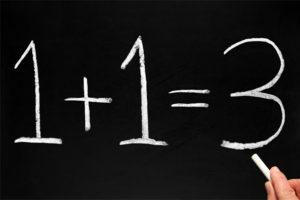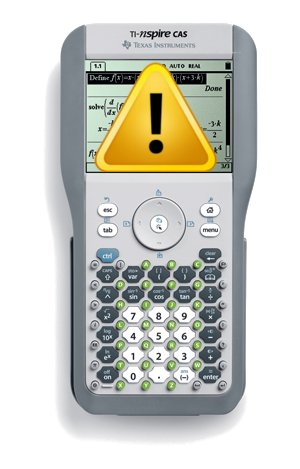Posted on

## Statistics and the “Scientific Method”# The “Scientific Method”

Most secondary school students are taught about the “Scientific Method” during their general science classes. From these early classes, students are encouraged to believe that all scientists follow an objective and almost linear process in their research. The process is generally described as something like this:

1. an unusual observation is made
2. an explanation is proposed for this observation
3. experiments are performed to test the explanation
4. after repeated experiments a theory is formed
5. experiments are performed to validate the theory
6. once validated the theory becomes a new scientific law
7. the scientific laws are used to predict new observations
Posted on

## Statistics in “APA Format”Mathematics is an ancient discipline, developed through many cultures and with many different ways of representing numbers and operations. Fortunately, there is a accepted convention that now governs how mathematics is represented around the world and it is through this convention that many mathematicians are able to exchange ideas independent of whether they speak the same (non-mathematical) language. It is not surprising, therefore, that statistics (a branch of mathematics) also has a convention on how statistics are represented.

## Statistical Conventions

In the statistics discipline we are typically dealing with one of two groups, the population or the sample. The population we are working with should be denoted by a capital Roman letter (e.g.$X$ ) and an individual from that population should be denoted with a lower case Roman letter (e.g.$x$ ). When we take a sample from the population, we are getting a set of$x$ ‘s out of our population$X$  (i.e each$x \in X$ )

Posted on

## Infallible Calculator?You may have seen the claim on the Internet that millions of people get the answer to the equation$6 \div 2\left(1 + 2\right)$  (or similar) wrong. You may have even read the explanations by some people about why the answer should be 9 or should be 1. Let me clear it up and explain why the answer is most definitely 1, but why the calculator (and others) give 9.

Firstly, the important thing to recognise is that this equation, like all others, is subject to the Order of Operations, which states that we must solve the contents of the brackets first. Thus our equation becomes$6 \div 2(3)$ .

Posted on

## Proof that 1 + 1 = 1. Not 2!?!Here is a quick demonstration of what can go wrong when you violate the rules of mathematics.

1. Let$a = 1$  and$b = 1$ .

Posted on

## The Secret to Success in MathematicsThere are so many people who struggle with mathematics and yet some people seem to have no trouble whatsoever. Is there something different in their DNA or some super advanced part of their brains, or do they simply know some secret trick that helps them in their understanding?

The answer is a little of the second, but mostly the last. Scientific research suggests that those who are good at mathematics do have some areas of their brain more active during mathematical activities, however, this increased brain activity could be a result of increased mathematical training. Much like how an athlete’s muscles improve with training, regular mathematical exercises help to improve the performance of those brain areas associated with mathematics. Again, like an athlete in training, there needs to be some underlying skills and a good coach. All of us possess the minimum underlying skills, but not all of us are fortunate enough to have a good coach at the critical time when we are ready to start serious mathematical training.